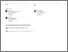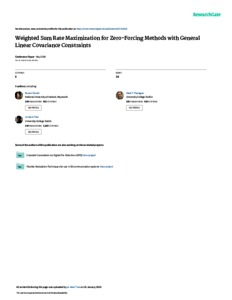# Weighted Sum Rate Maximization for Zero-forcing Methods with General Linear Covariance Constraints

Pham, Thuy M. and Farrell, Ronan and Claussen, Holger and Flanagan, Mark and Tran, Le-Nam (2018) Weighted Sum Rate Maximization for Zero-forcing Methods with General Linear Covariance Constraints. IEEE Xplore.Preview
Download (406kB) | Preview

Share your researchmore...Add this article to your Mendeley library

## Abstract

In this paper, an efﬁcient approach for weighted sum rate maximization (WSRMax) for zero-forcing(ZF)methods with general linear transmit covariance constraints (LTCCs) is proposed. This problem has been extensively studied separately for some special cases such as for sum power or per-antenna power constraints (PAPC). Due to some practical and regulatory requirements, these power constraints alone are not in general sufﬁcient, which motivates the consideration of general LTCCs. On the other hand, the zero-forcing (ZF) is a simple linear precoding technique to mitigate inter-user interference. The problem of WSRMax for ZF methods with LTCCs was studied previously using a gradient descent algorithm with barrier functions, but this method was also shown to converge slowly. To derive an efﬁcient solution to this problem, we ﬁrst reformulate it as an equivalent minimax problem using Lagrangian duality. The obtained result in fact resembles BC-MAC duality but is specialized for ZF methods. We then combine alternating optimization and concave-convex procedure to efﬁciently compute a saddle point of the minimax problem. The proposed method is numerically shown to converge very fast and its complexity scales linearly with the number of users.

Item Type: Article Weighted Sum Rate; Maximization; Zero-forcing Methods; General Linear; Covariance Constraints; Faculty of Science and Engineering > Computer Science 12685 Ronan Farrell 02 Apr 2020 10:39 IEEE Xplore IEEE Xplore Yes

### Repository Staff Only(login required)Item control page

### Downloads

Downloads per month over past year